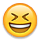# Battery bank question??

The question is more about capacity as related to voltage. To keep the math simple for a newb lets say 200 ah at 24 volts and then at 48 volts. Using four (6 volt 200 ah) batteries to equal 24 volts and eight to equal 48 volts the amp hours remain the same. How do I calculate the discharge rate assuming the loads are the same?

Dennis

• Solar Expert Posts: 5,183 ✭✭✭✭
Re: Battery bank question??

look at the watt-hrs of those banks and look at the watts used by the appliances and the watt-hrs on your battery monitor...

KID #51B  4s 140W to 24V 900Ah C&D AGM
CL#29032 FW 2126/ 2073/ 2133 175A E-Panel WBjr, 3 x 4s 140W to 24V 900Ah C&D AGM
Cotek ST1500W 24V Inverter,OmniCharge 3024,
2 x Cisco WRT54GL i/c DD-WRT Rtr & Bridge,
Eu3/2/1000i Gens, 1680W & E-Panel/WBjr to come, CL #647 asleep
• Solar Expert Posts: 3,123 ✭✭✭✭
Re: Battery bank question??
verdigo wrote: »
The question is more about capacity as related to voltage. To keep the math simple for a newb lets say 200 ah at 24 volts and then at 48 volts. Using four (6 volt 200 ah) batteries to equal 24 volts and eight to equal 48 volts the amp hours remain the same. How do I calculate the discharge rate assuming the loads are the same?

You have to assume that "the loads are the same" means that the loads get adjusted to draw the same power at 48 volts that they did at 24 volts, or else that the loads are powered by first a 24 volt and then a 48 volt inverter.

For the same wattage load, the battery current will be one half as much at 48 volts as it was at 24 volts. And you have the same AH of batteries, so they will last twice as long on a charge.
The same result as if you just say intuitively that you have twice as many identical batteries so they should last twice as long.
:-)
SMA SB 3000, old BP panels.
• Re: Battery bank question??
westbranch wrote: »
look at the watt-hrs of those banks and look at the watts used by the appliances and the watt-hrs on your battery monitor...

Thank you. That makes sense.
• Re: Battery bank question??